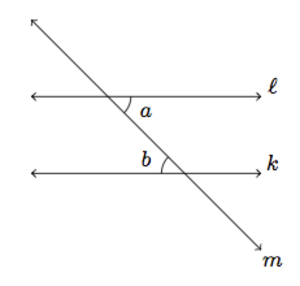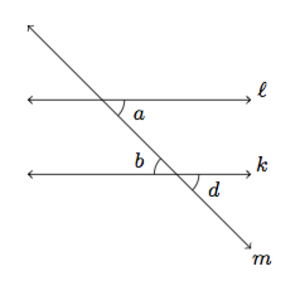# Rigid motions and congruent angles

Alignments to Content Standards: 8.G.A.5

In the picture below, $\ell$ and $k$ are parallel lines:1. Show that angle $a$ is congruent to angle $b$ using rigid motions.
2. Which other angles, made by the intersection of $\ell$ and $m$ or by the intersection of $k$ and $m$, are congruent to $a$? Explain using rigid motions.

## IM Commentary

The goal of this task is to use rigid motions to establish some fundamental results about angles made by intersecting lines. Both vertical angles and alternate interior angles are treated. This task is a decontextualized version of https://www.illustrativemathematics.org/illustrations/1936 and, as a result, the level of rigor expected in describing and applying rigid motions is higher. Students should already be comfortable with analyzing a situation with numerical angle measures before undertaking this task. Part (a) of the task is the content of https://www.illustrativemathematics.org/illustrations/1503.

If computer technology is available, it would be very helpful for students to help visualize the different transformations which they perform. Patty paper would be another good alternative. The teacher may wish to provide the parallel lines $\ell$ and $k$ only and have students draw in a transverse $m$. Then students can be prompted to identify a pair of alternate interior angles and show, with rigid motions, that they are congruent. If students happen to draw a perpendicular transverse, then they will be showing that when a line $M$ is perpendicular to a line $L$, it is also perpendicular to any line $K$ parallel to $L$.

## Solution

1. We can first translate $\ell$ along $m$ so that the intersection of $m$ and $\ell$ moves to the intersection of $m$ and $k$. Since $\ell$ and $k$ were parallel, this translation moves $\ell$ onto $k$, and so $a$ is congruent to its image, $d$, marked in the picture below:We can now apply a 180-degree rotation about the intersection of $k$ and $m$. After these two transformations, angle $a$ is mapped to angle $b$, showing that these two angles are congruent. An alternative way to show that $a$ and $b$ are congruent is sketched below.

In the picture below, the intersection of $\ell$ and $m$ is labeled $P$, the intersection of $k$ and $m$ is labeled $Q$ and $R$ is the midpoint of $\overline{PQ}$:If we rotate the plane about $R$ by 180 degrees, this maps $R$ to itself and it sends $P$ to $Q$ and $Q$ to $P$. Rotation by 180 degrees about $R$ also takes $\ell$ to $k$ and $k$ to $\ell$ and it exchanges the angles marked $a$ and $b$. Rotations preserve angle measure so this means that angles $a$ and $b$ are congruent.

2. The picture below shows the angles which are congruent to $a$:We have already seen that $a$ and $b$ are congruent. Angles $a$ and $c$ make a vertical pair so they are also congruent (as can be shown with a 180 degree rotation about $P$). Angles $b$ and $d$ are vertical angles and so they are also congruent: since $a$ and $b$ are congruent, this means that $a$ is congruent to $d$. The other angles made by the intersection of these lines are all obtuse (or measure more than 180 degrees) and so are not congruent to angle $a$.